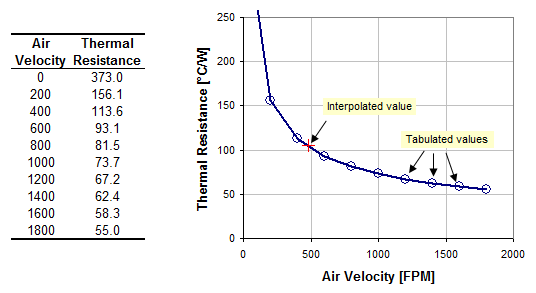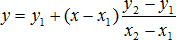Many people want to interpolate data they have digitized with Dagra in Microsoft Excel. Unfortunately Excel doesn’t provide an interpolation function but there is a simple approach.

### Understanding Interpolation

Interpolation is a method for estimating the value of a function between two known values. Often some relationship is measured experimentally or traced with Dagra at a range of values. Interpolation can be used to estimate the function for untabulated points.

For example, suppose we have tabulated data for the thermal resistance of a transistor tabulated for air velocity from 0 to 1800 FPM in 200 FPM steps. Interpolation can be used to estimate the thermal resistance at non-tabulated values such as 485 FPM.The table lists thermal resistance in 200 FPM steps. Interpolation can be used to estimate the thermal resistance for non-tabulated values between the tabulated ones.

### Linear Interpolation Equations

Linear interpolation involves estimating a new value by connecting two adjacent known values with a straight line.

If the two known values are (x1, y1) and (x2, y2), then the y value for some point x is:### Implementing Interpolation with Microsoft Excel

The linear interpolation equation above can be implemented directly in Microsoft Excel provided the tabulated values are monotonic in x, that is the x-values are sorted and no two are equal. The online Microwave Encyclopedia has the full 6 line implementation along with a good explanation of how it works.

However, here is a simpler implementation for Excel:
`=FORECAST(NewX,OFFSET(KnownY,MATCH(NewX,KnownX,1)-1,0,2), OFFSET(KnownX,MATCH(NewX,KnownX,1)-1,0,2))`
To use it either:

1. Copy the formula above into Excel and replace KnownX and KnownY with the cell reference for the tabulated x and y values and NewX with the x-value to interpolate, OR
2. Define names for the KnownX and KnownY ranges ( in Excel 2003) and replace NewX with the x-value to interpolate.

LinearInterpolation
LinearInterpolation.xls
21.0 KiB
Details

This equation works exactly the same way as the direct implementation of the linear interpolation equation. The main difference is that only two lookup functions are required for the simple approach described here, while the direct implementation needs 6 (one for each term in the equation). This makes it substantially faster.

### How the Excel implementation works

The simple implementation is easiest to understand by dissecting from the outside and working in. Here’s the full equation:
`=FORECAST(NewX,OFFSET(KnownY,MATCH(NewX,KnownX,1)-1,0,2), OFFSET(KnownX,MATCH(NewX,KnownX,1)-1,0,2))`
In brief, the equation consists of 3 parts:

1. the FORECAST function to calculate the linear interpolation,
2. two calls to the MATCH function to find the tabulated x-value closest too, but less than the new-x value, and
3. two calls to the OFFSET function to reference the tabulated x-values and y-values just above and just below the new-x value.

In more detail, the FORECAST function performs the actual interpolation using the linear interpolation equation shown above. Its syntax is: FORECAST(NewX, known_y_pair, known_x_pair).

The first parameter, NewX is simply the value to interpolate. The next two parameters, known_y_pair and known_x_pair are the values either side of NewX. That is, {x1, x2} and {y1, y2} in the diagram above.

The MATCH function is used to find the tabulated x-value just below NewX. Its syntax is: MATCH(lookup_value, lookup_table,match_type). MATCH returns the relative position of an item in a sorted array. So, lookup_value is the value to interpolate, lookup_table is the array of KnownX values, and match_type is 1 to find the largest value in the array that is less than or equal to NewX.

The MATCH function returns an index, but the FORECAST function requires two cell ranges: one for the known_x_pair and one for the known_y_pair. So, the OFFSET function is used twice to create these ranges. Its syntax is OFFSET(reference,row_offset, column_offset, row_count,column_count). It takes a starting point, the reference, and creates a cell reference with the given offset and size. To obtain the known_y_pair range, the reference is set to the table of KnownYvalues; for the known_x_pair range, reference is set to the array of KnownX values. If the tabulated values are arranged vertically, the row_offset is the result from the MATCH function less 1 and row_count is 2; column_offset is 0 andcolumn_count is 1. This gives us a cell array reference 2 cells high and 1 cell wide. If the tabulated values are arranged horizontally, row and column are switched in the OFFSET function.

Not readable? Change text.